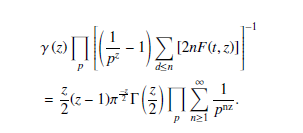# The Derivation of the Riemann Analytic Continuation Formula from the Euler’s Quadratic Equation

## Authors

• Opeyemi O. Enoch Department of Mathematics, Federal University Oye-Ekiti, Ekiti State, Nigeria
• Adejimi A. Adeniji Department of Mathematics and Statistics, Tshwane University of Technology, South Africa
• Lukman O. Salaudeen Department of Mathematics, Federal University Oye-Ekiti, Ekiti State, Nigeria

## Keywords:

Riemann zeta function, Euler’s equation, Meromorphic function, Non-trivial zeros

## Abstract

The analysis of the derivation of the Riemann Analytic Continuation Formula from Euler’s Quadratic Equation is presented in this paper. The connections between the roots of Euler’s quadratic equation and the Analytic Continuation Formula of the Riemann Zeta equation are also considered. The method of partial summation is applied twice on the resulting series, thus leading to the Riemann Analytic Continuation Formula. A polynomial approach is anticipated to prove the Riemann hypothesis; thus, a general equation for the zeros of the Analytic Continuation Formula of the Riemann Zeta equation based on a polynomial function is also obtained. An expression in Terms of Prime numbers and their products is considered and obtained. A quadratic function, G(tn), that is required for Euler’s quadratic equation (EQE) to give the Analytic Continuation Formula of the Riemann Zeta equation (ACF) is presented. This function thus allows a new way of defining the Analytic Continuation Formula of the Riemann Zeta equation (ACF) via this equivalent equation. By and large, the Riemann Zeta function is shown to be a type of L function whose solutions are connected to some algebraic functions. These algebraic functions are shown and presented to be connected to some polynomials. These Polynomials are also shown to be some of the algebraic functions’ solutions. Conclusively, &(z) is redefined as the product of a new function which is called H(tn; z) and this new function is shown to be dependent on the polynomial function, G(tn).

Dimensions

M. Griffin, K. Ono, L. Rolen, & D. Zagier, “Jensen polynomials for the riemann zeta function and other sequences”, Proceedings of the National Academy of Sciences 116 (2019) 11103.

D. K. Dimitrov & Y. B. Cheikh, “Laguerre polynomials as jensen polynomials of laguerre–p´olya entire functions”, Journal of computational and applied mathematics 233 (2009) 703.

S. DeSalvo & I. Pak, “Log-concavity of the partition function”, The Ramanujan Journal 38 (2015) 61.

J. Derbyshire, Prime obsession: Bernhard Riemann and the greatest unsolved problem in mathematics, Joseph Henry Press (2003).

O. O. Enoch, “The eigenvalues (energy levels) of the riemann zeta function”, International Conference on Pure and Applied Mathematics (2015) 81.

O. O. Enoch, “The derivation of the riemann zeta function from euler’s quadratic equation and the proof of the Riemann hypothesis”, International Scientific Journal. Journal of Mathematics (2016).

O. O. Enoch, “From the zeros of the Riemann zeta function to its analytical continuation formula”, Global Journal of Pure and Applied Mathematics 13 (2017) 8423.

M. Chudnovsky & P. Seymour, “The roots of the independence polynomial of a claw free graph”, Journal of Combinatorial Theory, Series B 97 (2007) 350.

H. Larson & I. Wagner, “Hyperbolicity of the partition jensen polynomials”, Research in Number Theory 5 (2019) 1.

A. Torres-Hernandez & F. Brambila-Paz, “An approximation to zeros of the riemann zeta function using fractional calculus”, arXiv preprint arXiv:2006 (2020) 14963.

A. Selberg, “Harmonic analysis and discontinuous groups in weakly symmetric riemannian spaces with applications to dirichlet series”, The Journal of the Indian Mathematical Society 20 (1956) 47.

S. Andreas, Riemann’s second proof of the analytic continuation of the riemann zeta function (1987).

R. R. Ben, The zeta function and its relation to the prime number theorem (2000).

S. J. Patterson, “An introduction to the theory of the Riemann zetafunctio”, Cambridge University Press 14 (1995).

A. Selberg, “An elementary proof of the prime number theorem”, Annals of Mathematics (1949) 305.

O. O. Enoch, T. O. Ewumi, & Y. Skwame, “On the turning point, critical line and the zeros of riemann zeta function”, Australian Journal of Basic and Applied Sciences 6 (2012) 279.2022-12-20

## How to Cite

The Derivation of the Riemann Analytic Continuation Formula from the Euler’s Quadratic Equation. (2022). Journal of the Nigerian Society of Physical Sciences, 5(1), 967. https://doi.org/10.46481/jnsps.2023.967

## Section

Original Research

## How to Cite

The Derivation of the Riemann Analytic Continuation Formula from the Euler’s Quadratic Equation. (2022). Journal of the Nigerian Society of Physical Sciences, 5(1), 967. https://doi.org/10.46481/jnsps.2023.967